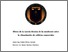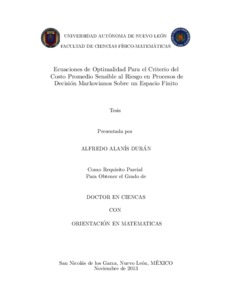# Ecuaciones de optimalidad para el criterio del costo promedio sensible al riesgo en procesos de decisión markovianos sobre un espacio finito

Alanís Durán, Alfredo (2013) Ecuaciones de optimalidad para el criterio del costo promedio sensible al riesgo en procesos de decisión markovianos sobre un espacio finito. Doctorado thesis, Universidad Autónoma de Nuevo León.Vista previa
Texto

## Resumen

This work concerns Markov decision chains endowed with the risk-sensitive average cost criterion, and the main goals are to characterize the optimal value function and to determine an optimal stationary policy. The exposition begins in Chapter 1 where the notion of Markov decision chain is introduced, and the ideas of risk-aversion and risk–sensitivity coefficient are briefly discussed. After this point, the risk-sensitive average cost criterion is formulated, and the main objectives are formally stated. Next, in Chapter 2 a fundamental theorem by Howard and Matheson (1972), as well as a recent extension on the characterization of the optimal average cost in terms of a single optimality equation are analyzed; such results require that, under the action of any stationary policy, every state can be visited with positive probability regardless of the initial sate, and the arguments used in this work emphasize the central role of that communication property. The presentation continues in Chapter 3 studying a recent theorem on the existence of solutions to the optimality equation for ‘small’values of the risk-sensitivity coefficient, which was derived under the assumption that there exists a state that can be always reached with positive probability under the action of any stationary policy; the derivation presented in this work highlights the fundamental role of such an accessibility condition. The conclusions in Chapters 2 and 3 provide the motivation to pursue the main objective of this thesis, namely, to establish a characterization of the optimal risk-sensitive average cost without imposing any condition on the structure of the transition law of the model. This goal is achieved in Chapter 4, where the optimal risk-sensitive average cost function is characterized for general controlled Markov chains with finite state space and compact action sets, a result that is the main contribution of this thesis. It is supposed that the decision maker is risk-averse with constant risk-sensitivity coefficient and, under standard continuity–compactness conditions, it is proved that the (possibly non-constant) optimal value function is characterized by a nested system of equations, generalizing the conclusions s presented in the previous chapters, which require communication conditions on the transition law; moreover, it is shown that an optimal stationary policy can be derived form a solution of that system, and that the optimal superior and inferior limit average cost functions coincide. The approach used to obtain the main conclusions relies on the discounted method which, roughly, consists in using a family of contractive operators whose fixed points are used to approximate the optimal average index, to partition the state space in a family of equivalence classes, to determine a class of admissible actions at each state, and to construct a solution of a ‘reduced’ optimality equation on each equivalence class; the presentation of these results is based on the recent paper Alanís Durán and Cavazos-Cadena (2012). Finally, the exposition concludes in Chapter 5 with a retrospective view of the material presented in this work, and with the statement of two open problems concerning the extension of some of the conclusions in this work to models with denumerable state space.

Información adicional: Doctor en ciencias con orientación en matemáticas
Divisiones: Ciencias Físico MatemáticasVer elemento